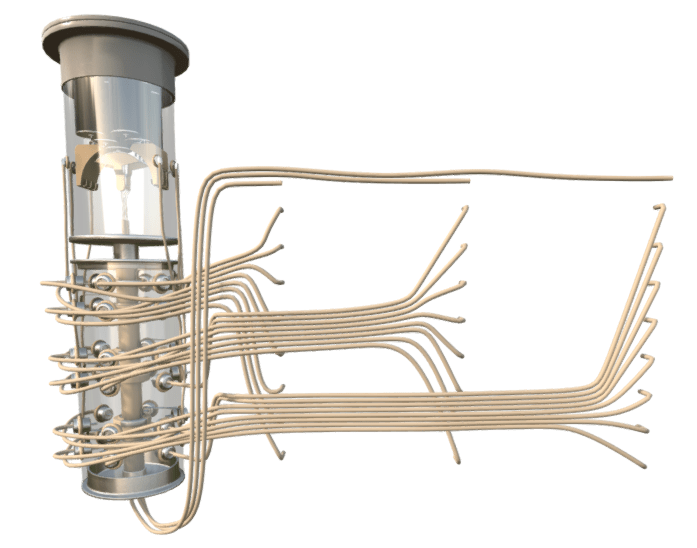# On Load Tap Changer (LTC)

### Introduction

On-load tap changers (LTC, or, OLTC) regulate the turns ratio and thus the voltage ratio of an electrical transformer. Unlike its no-load counterpart, on-load tap changers do this without interrupting the load current. There are two main load tap changer designs, the reactance and the resistive types. Load tap changers are often employed with conservator type transformers, but seldom with hermetic transformers.An LTC (the casing is not normally transparent)

The amount an LTC can regulate the output voltage varies, although a typical range is ±10% of rated output voltage. For large distribution transformers, it is typical to have 16 taps above and 16 taps below the rated voltage. Each tap adjusts the voltage by 0.625% until it is either a maximum of 10% above rated voltage or 10% below rated voltage.

### Example

For a transformer with a rated output of 100,000V, a tap movement of three positions to increase the voltage represents a total voltage increase of 1.875%. The new output voltage is thus 101,875V because:

100,000V + ((100,000V x (0.625% x 3)) = 100,000V + 1,875V = 101,875V

Where:

Secondary Voltage = 100,000V

Tap Movement = 0.625 x 3 = 1.875%

## How do on-load tap changers work?

The below video is an extract from our Introduction to Electrical Transformers Online Video Course.